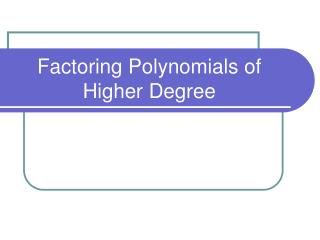DownloadDownload PresentationFactoring Polynomials of Higher Degree

# Factoring Polynomials of Higher Degree

Télécharger la présentation## Factoring Polynomials of Higher Degree

- - - - - - - - - - - - - - - - - - - - - - - - - - - E N D - - - - - - - - - - - - - - - - - - - - - - - - - - -
##### Presentation Transcript

1. Factoring Polynomials of Higher Degree

2. Factoring Polynomials of Higher Degree • To review: What is the remainder when you divide x3 – 4x2 – 7x + 10 by x – 2? Quotient Divisor Dividend Remainder

3. Factoring Polynomials of Higher Degree Remainder Theorem: If a polynomial p(x) is divided by the binomial x – a, the remainder obtained is p(a). So, looking at our example, if p(x) = x3 – 4x2 – 7x + 10 was divided by x – 2, the remainder can be determined by finding p(2). p(x) = x3 – 4x2 – 7x + 10 p(2) = (2)3 – 4(2)2 – 7(2) + 10 = 8 – 16 – 14 + 10 = -12

4. Factoring Polynomials of Higher Degree The Factor Theorem (Part 1) If p(a) = 0, then x – a is a factor of p(x) So let’s see what happens if p(1). p(1) = (1)3 – 4(1)2 – 7(1) + 10 = 1 – 4 – 7 + 10 = 0  Since p(1) = 0, we know that x – 1 is also a factor of p(x).

5. Factoring Polynomials of Higher Degree Example 1: Determine the remainder when p(x) = x3 + 5x2 – 9x – 6 is divided by x – 3. Solution: When p(x) is divided by x – 3, the remainder is p(3). p(3) = 33 + 5(3)2 – 9(3) – 6 = 27 + 45 – 37 – 6 = 39 Thus, the remainder is 39. Since the remainder is not 0, x – 3 is not a factor of p(x).

6. Factoring Polynomials of Higher Degree • In order to assist with the factoring of higher order polynomials, we use a process known as synthetic division. • A quick process to divide polynomials by binomials of the form x – a and bx – a.

7. Factoring Polynomials of Higher Degree • Looking at our example: • Synthetic Division • Create a “L” shape • Place “a” toward the upper left of the “L” • Record the coefficients of the polynomials inside the “L” 2 1 -4 -7 10 Write in decreasing degree and 0’s need to be recorded as coefficients for any missing terms.

8. Factoring Polynomials of Higher Degree Synthetic Division 4) Bring down 1 5) Multiply 1 by 2 and record result under – 4 6) Add -4 to 2 and record answer below the “L” 7) Repeat process until you reach the last term 8) The number on the furthest right below the “L” is the remainder. 2 1 -4 -7 10 2 -4 -22 1 -2 -11 -12 Remember that 1 is the number of x2’s, -2 is the number of x’s, and -11 is the constant term of the quotient polynomial.

9. Factoring Polynomials of Higher Degree Example 2: Use synthetic division to find the quotient and remainder when x3 – 4x2 – 7x + 10 is divided by x – 5. 5 1 -4 -7 10 5 5 -10 Remainder 0 means that x – 5 is a factor of p(x) 1 1 -2 0

10. Homework • Do # 1, 3, 5, 7, 9, 17, 18, 21 on pg 130 in Section 4.3 for Monday  Have a great weekend!!!